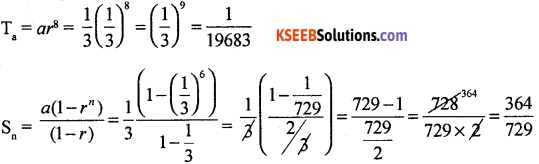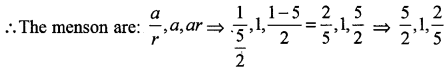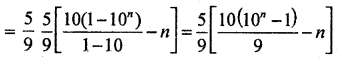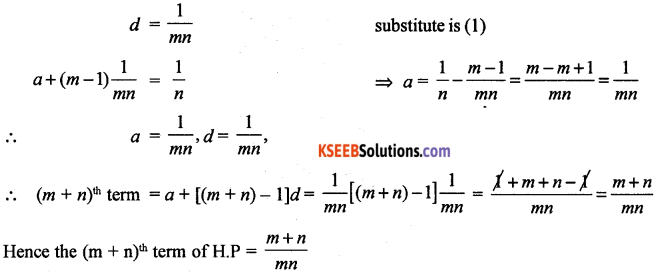# 1st PUC Basic Maths Question Bank Chapter 5 Progressions

Students can Download Basic Maths Chapter 5 Progressions Questions and Answers, Notes Pdf, 1st PUC Basic Maths Question Bank with Answers helps you to revise the complete Karnataka State Board Syllabus and score more marks in your examinations.

## Karnataka 1st PUC Basic Maths Question Bank Chapter 5 Progressions

Arithmetic Progression (A.P)

Question 1.
Which term of A.P 4$$\left(5 \frac{1}{3}-4\right), 6 \frac{2}{3} \ldots \ldots$$ is 104?
In given A.P: a = 4, d = 51/3 – 4 = $$\frac { 16 }{ 3 }$$ – 4 = $$\frac { 4 }{ 3 }$$
Let nth term be 104 = Tn = a + (n – 1) d
⇒ 4 + (n – 1) $$\frac { 4 }{ 3 }$$ = 104
⇒ 12 + 4n – 4 = 312 ⇒ 4n – 4 = 312
⇒ 4n = 312 – 8
⇒ 4n = 304
∴ n = 76

Question 2.
How many terms of series 24 + 20 + 16 + ……. must be taken so that their sum is 72.
In the given A.P; a = 24, d = 4, Sn = 72
we have Sn = $$\frac { n }{ 2 }$${2a + (n – 1)d}
72 = $$\frac { n }{ 2 }$${2(24) + (n – 1)(-4)}
= 144 = n{48 – 4n + 4}
⇒ 144 = n{52 – 4n}
⇒ 144 = 52n – 4n2
⇒ 4n2 – 52n + 144 = 0
⇒ n2 – 13n + 36 = 0
⇒ (n – 9)(n – 4) = 0
∴ n = 9,4Question 3.
The first and the last term of an A.P are -4 and 146 and the sum of the A.P is 7171. Find the number of terms in the A.P and the A.P.
Given a = -4, l = 146.
We have l = a + (n – 1 )d = 146 = -4 + (n – 1) d
⇒ (n – 1)d = 150
Also we have Sn = $$\frac { n }{ 2 }$${2a + (n – 1)d}
7171 = $$\frac { n }{ 2 }$${2(-4) + 150}
14342 = n{-8 + 150}
⇒ 142n = 14342
∴ n = $$\frac{14342}{142}$$ = 101
So number of terms is A.P = 101
consider (n – 1)d = 150.Question 4.
Find the sum of all numbers between 100 and 1000 which are divisible by 11.
The 1st greatest No. greater than 100 is 110. the last which is less than 1000 is, 990.
110,121, ………990 be the number
Tn = 90 ⇒ 110 + (n – 1) 11 = 990
11n – 11 = 990 – 110
11n = 880 + 11 = 891
∴ n = $$\frac { 891 }{ 11 }$$ = 81
Now S81 = 8$$\frac { 1 }{ 2 }$$(110 + 990) = $$\frac { 81 }{ 2 }$$(550) = 4450

Question 5.
The first, second and the last terms of an A.P are a, b, c respectively prove that the sum is $$\frac{(a+c)(b+c-2 a)}{2(b-a)}$$
1st term is a, common difference = d = b – a.
Let ‘n’ be the number of term in the series.
∴ c = a + (n – 1)d
⇒ (n – 1)d = c – a
⇒ (n – 1 )(b – a) = c – a
⇒ n – 1 = $$\frac{c-a}{b-a}$$
⇒ n = $$\frac{c-a}{b-a}$$ + 1
= $$\frac{n}{2}$${2a + c – a} = $$\frac{n}{2}$${a + c} = $$\left(\frac{b+c-2 a}{b-a}\right)\left(\frac{a+c}{2}\right)$$

Geometric Progression (G.P)

Question 1.
Find the 9th term and sum of 6 term of the geometric sequence $$\frac{1}{3}, \frac{1}{9}, \frac{1}{27} \ldots \ldots$$
a = $$\frac{1}{3}$$ r = $$\frac{1}{3}$$Question 2.
The second and fifth term of GP are 3 and $$\frac{81}{8}$$ respectively. Find the GP
Given T2 = ar = 3, T5 = ar4 = $$\frac{81}{8}$$∴ r = $$\sqrt{\frac{33}{23}}=\frac{3}{2}$$
If ar = 3 ⇒ a. $$\frac{3}{2}$$ = 3 ⇒ a = 2
G.p is 2, 3, $$\frac{9}{2}$$, …….

Question 3.
The sum of three numbers which are in G.P is $$\frac{39}{10}$$ and their product is 1. Find the numbers.
Let the number be $$\frac{a}{2}$$, a, ar. which are in G.P.
i.e., $$\frac{a}{2}$$ + a + ar = $$\frac{39}{10}$$⇒ a3 = 1
∴ a = 1
put a = 1 in (1),
$$\frac{1}{r}$$ + 1 + r = $$\frac{39}{10}$$
⇒ $$\frac{1+r+r^{2}}{r}=\frac{39}{10}$$
⇒ 10 + 10r + 10r3 = 39
r ⇒ 10r2 – 29r + 10 = 0
⇒ r = $$\frac{5}{2}$$ and $$\frac{2}{5}$$Question 4.
Find three numbers in GP. Whose sum is 35 and sum of whose squares is 525.
Let the three numbers be a, ar, ar2 which are is GP.
Given a + ar + ar2 = 35 ⇒ a( 1 + r + r2) = 35
and a2 + a2r2 + a2r4 = 525
⇒ a2( 1 + r2 + r4) = 525
$$\frac{a^{2}\left(1+r^{2}+r^{4}\right)}{a\left(1+r+r^{2}\right)}=\frac{525}{35}=15$$
a(1 + r2 + r4) = 15(1 + r + r2)⇒ a(1 – r + r2) = 15 …….. (2)
2a + 2ar2 = 50
all (2) – (1) ⇒ 2ar = -20$$\frac{1}{r}$$ + r = $$\frac{-5}{2}$$
⇒ 2(1 + r2) = -5r
⇒ 2r2 + 5r + 2 = 0
⇒ r = $$\frac{-5 \pm \sqrt{25-4(2)(2)}}{4}$$
⇒ $$\frac{-5 \pm 3}{4}=\frac{-1}{2},-2$$
when r = -2 2ar = -20 ⇒ -4a = -20 [∴ a = 5]
when r = $$-\frac{1}{2}$$ , 2ar = -20 ⇒ -a = -20 ⇒ [a = 20]
Hence the three number which are in G.P are 5, -10, 20 (or) 20, – 10, 5

Question 5.
Find the sum of n terms of 5 + 55 + 555 + ……..
Let s = 5 + 55 + 555 + …….. n terms
⇒ s = 5[1 + 11 + 111 + …… to term
= $$\frac{5}{9}$$(9 + 99 + 999 + ….. to n term) $$\frac{5}{9}$$[(10 – 1) + (100 – 1) + (1000 – 1) + …… to terms]
= $$\frac{5}{9}$$[10 + 102 + 103 + …… to n terms] + (1 + 1 + ……. to n terms)Harmonic Progression (H.P)

Question 1.
If the 12th element of H.P is $$\frac { 1 }{ 5 }$$ and the 19th term is $$\frac { 3 }{ 32 }$$. Find the 10th term of H.P.
12th term = $$\frac { 1 }{ 15 }$$
19th term = $$\frac{3}{2^{2}}$$
∴ 12th term, AP is 5 and
19th termof A.P is $$\frac { 22 }{ 3 }$$Question 2.
If 1, x, 3 are in H.P . Find x.
Given 1, x, 3 are in H.PQuestion 3.
If the mth term of H.P is ‘n’ and nth term is m, prove that (m + n)th is $$\frac{m n}{m+n}$$
Given mth term of H.P is n,
∴ mth term of A.P = $$\frac{1}{n}$$ = a + (m – 1) d
Also nth term of HP is m.
∴ nth term of A.P = $$\frac{1}{m}$$ = a + (n – 1)d
a + (m – 1)d = $$\frac{1}{n}$$ …… (1)
a + (n – 1)d = $$\frac{1}{m}$$ …… (2)
Solving (1) and (2)Arithmetic, Geometric and Hormonic Means.

Question 1.
Find six geometrical mean between 27 and $$\frac { 1 }{ 81 }$$
Let the required GM is g1, g2, g3, g4, g5, g6
= 27, g1, g2, g3, g4, g5, g6, $$\frac{1}{81}$$ are in GP
Now a = 27 and $$\frac{1}{81}$$ = 8th element
⇒ $$\frac{1}{81}$$ = ar7 ⇒ $$\frac{1}{81}$$ = 27.r7
⇒ $$\frac{1}{81 \times 27}$$ = r7
⇒ r7 = $$\frac{1}{3^{7}}$$
⇒ r = $$\frac{1}{3}$$
∴ the six GM are 9, 3, 1,$$\frac{1}{3}, \frac{1}{9}, \frac{1}{27}$$

Question 2.
If a, b, c are in A.P and a, mb, c are in GP then show that a, m2b, c are in H.P
a, b, c, are in AP .
⇒ b = $$\frac{a+c}{2}$$
a, mb, c are in GP,
⇒ mb = $$\sqrt{a c}$$
⇒ m2b2 = ac
⇒ m2b. b. = ac
⇒ m2b $$\left(\frac{a+c}{2}\right)$$ = ac $$\left(\because b=\frac{a+c}{2}\right)$$
⇒ m2b = $$\frac{2 a c}{a+c}$$
⇒ a, m2b, c are in H.P.Summation of Series

Question 1.
12 + 32 + 52 + ………  to ’n’ terms.
1, 3, 5, ………. are in A.P
Tn = a + (n – 1)d = 1 + (n – 1)2 = 1 + 2x – 2 = 2x – 1
∴ nth term = (2n – 1)2
Tn = (2x – 1)2 = 4n2 – 4x + 1
required sum = Sn = ΣTn = Σ4n2 – 4x + 1 = 4Σn2 – 4Σn + Σ1.
$$=\frac{4 n(n+1)(2 n+1)}{6}-\frac{4 n(n+1)}{2}+n$$
= $$\frac{n}{3}$$ [2(n + 1)(2n + 1) – 6(n + 1) + 3] = $$\frac{n}{3}$$[4n2 – 1]

Question 2.
Find the sum to ‘n’ terms the series 1.2 + 4.5 + 7.8 + ….. to term.
1st factors are 1, 4, 7,
∴ nthterm = 1 + (n – 1) 3 = 3n – 2
2nd factor are 2, 5, 8,
∴ nth term = 2 + (n – 1) 3 = 3n – 1
⇒ nth term of the series is (3n – 2)(3n – 1)
∴ The sum = Σ(3n – 2)(3n – 1)
= Σ(9x2 – 9x + 2) = 9Σn2 -9Σn + 2Σ1
$$=9 \frac{n(n+1)(2 n+1)}{6}-9 \frac{n(n+1)}{2}+2 n$$
= $$\frac{1}{2}$$n(n + 1)[3(2n + 1) – 9] + 2n = n(3n2 – 1).Question 3.
Sum the series $$\frac{1^{3}}{1}+\frac{1^{3}+2^{3}}{1+3}+\frac{1^{3}+2^{3}+3^{3}}{1+3+5}+\ldots \ldots .16 \text { terms }$$ 16terms